# Intuitive Deep Learning Part 2: CNNs for Computer Vision

Source: Deep Learning on Medium

Where we left off: In part 1, we had an introduction to neural networks and how to make them work. We started off in Part 1a with a high-level summary of what Machine Learning aims to do:

Machine Learning specifies a template and finds the best parameters for that template instead of hard-coding the parameters ourselves. A neural network is simply a complicated ‘template’ we specify which turns out to have the flexibility to model many complicated relationships between input and output. Specifying a loss function and performing gradient descent helps find the parameters that give the best predictions for our training set.

We then dived into some nitty-gritty details in Part 1b:

Overfitting occurs when our model has fitted so well to the training dataset that it has failed to generalize to unseen examples. This is characterized by a high dev loss and a low train loss, and can be addressed with regularization techniques. The best model is the one that performed the best on our validation set. We can experiment with different hyper-parameters to specify different templates that can give us a lower validation loss. When we do gradient descent, we want to initialize the parameters with some randomness to break the symmetry. There is also another method called mini-batch gradient descent, which helps us achieve faster updates as it does not calculate the loss over all training examples.

In this post, we will turn our attention from the nitty-gritty of implementing Machine Learning models to the application of Machine Learning models to the domain of images. We can tweak the model architecture to be able to exploit properties of images — in particular, we will introduce a new model architecture called Convolutional Neural Networks (which are also called CNNs or ConvNets). The other parts about optimization largely remain unchanged from what we’ve discussed previously.

First, we need to talk about the properties of images before we can discuss how to exploit them in our neural network. In a computer, images are stored as a 2-dimensional array of pixels arranged spatially, each pixel corresponding to one small part of the image. In fact, the word “pixel” comes from the phrase “pi(x)cture element”, since it forms one small element within the image: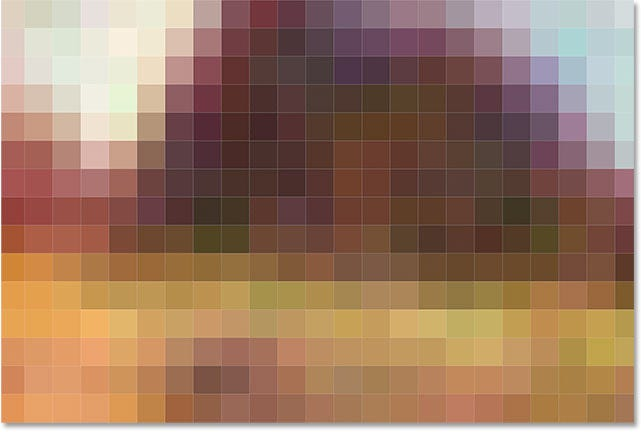Images are made out of pixels arranged within a 2-D array. Image taken from https://www.photoshopessentials.com/basics/pixels-image-size-resolution-photoshop/

Now each pixel stores a color; and a color is represented in a computer with three numbers, corresponding to the amount of Red, Green and Blue respectively in that color as a range from 0–255 (inclusive). So really, if you consider the color channels as another dimension, an image can be represented as a 3-dimensional array (the first two dimensions store the pixels as they are presented in the image, the last dimension has three channels — Red, Green and Blue).

What does this mean for our Deep Learning algorithms? When we take an image as an input, we are really taking a 3-D array of numbers. Each number is an individual feature of our image that we pass through our neural network. Suppose our image dimensions are 256 * 256; then we feed into our neural network as input 256 * 256 * 3 = 196,608 features in total. (That’s a lot of features!) And from these 196,608 features, we need to find some complicated function that transforms it into perhaps a prediction on what object the image represents. A simple example would be — is there a cat in the picture?

Recall that having 196,908 features means that we need 196,908 + 1 = 196,909 parameters in one neuron. Remember that our output of the neuron first takes some linear combination of the input features before applying an activation function: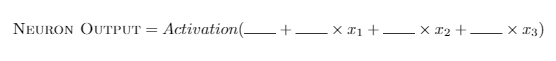A formulation of the neuron output in terms of its input features x1, x2 and x3

In the case of three input features (x1, x2 and x3), we have four blanks that we need to fill in the numbers to — one corresponding to each feature and one bias term (not attached to any feature). If we had 196,908 features, we are left with 196,909 blanks that we need to find the best numbers to. All of this in just one neuron! Now that’s a lot of parameters to learn!

To make things more complicated, the cat can be anywhere in the picture. The cat can be at the top right of the picture, or at the bottom left — they correspond to a very different set of 196,608 features yet they represent the same thing: a cat.

Summary: Images are 3-dimensional array of features: each pixel in the 2-D space contain three numbers from 0–255 (inclusive) corresponding to the Red, Green and Blue channels. Often, image data contains a lot of input features.

Recall from above that the nature of images are such that

1. There are a lot of ‘input features’, each corresponding to the R, G and B value of each pixel, which thus requires a lot of parameters.
2. A cat in the top left or a cat in the bottom right of the image should give similar outputs.

At this point, perhaps we can consider this following method. Suppose we have an image we want to test: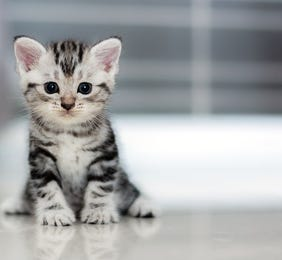An image of size 256×256 we wish to test whether there is a cat or not

Here is our algorithm:

Step 1: Split the image into four equal quadrants. Let’s take the image size to originally be 256 * 256 * 3(channels). Then, each quadrant of the image will have 128 * 128 * 3 features.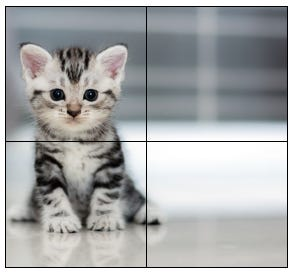We cut up our image into four equal quadrants of size 128×128 pixels. Remember that each pixel has 3 channels (R, G and B)

Step 2: Apply a neuron for the top-left quadrant to convert the 128 * 128 * 3 features into one single number. Just for intuition’s sake (although this is not entirely accurate), let’s say this neuron is in charge of recognizing a cat within the 128 * 128 * 3 features: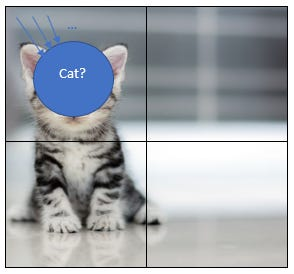We apply the neuron which takes in the 128 * 128 * 3 features in the top-left quadrant

Step 3: Apply the exact same neuron for the top-right quadrant, the bottom-left quadrant and the bottom-right quadrant. This is called parameter sharing, since we use the exact same neuron for all four quadrants.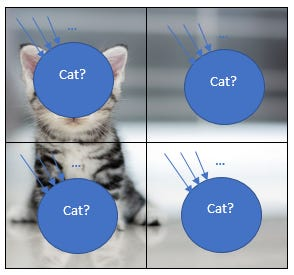We apply the exact same neuron with the exact same parameters to all four quadrants of the image

Step 4: After applying that neuron for all four quadrants, we have four different numbers (intuitively speaking, these numbers represent whether there is a cat or not in each of the quadrants).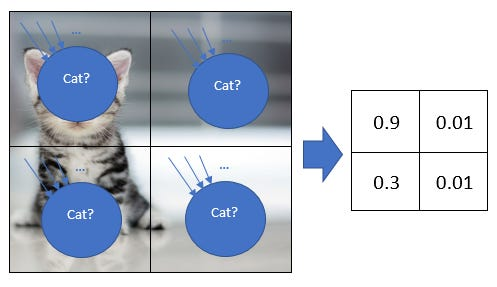We get four different output numbers even though we apply the same neuron since the inputs are different (although the parameters are the same)

Remember that we get four different numbers because we put different input features, even though the function (and the parameters remain the same).A formulation of the neuron output in terms of its input features x1, x2 and x3

From the above formulation, the input features (x1, x2 and x3) have changed even though the numbers filling in the blanks have not; therefore, these input features give rise to a different output.

Step 5: We want one number to tell us whether there is a cat in the entire picture. So we just take the maximum of those four numbers to get a single number.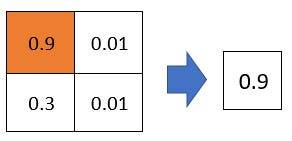We take the maximum of the four numbers to get one number in the end

What does this algorithm do in terms of addressing our earlier concerns?

• Our initial concern was that there were too many features and therefore too many parameters. Recall that even if we just have one neuron for all these features, we’d need 256 * 256 * 3 + 1 = 196,609 parameters for each neuron. If we split this into four different quadrants and use the exact same parameters for all four quadrants, we only need 128 * 128 * 3 + 1 = 49,153 parameters — a reduction by almost four times!
• It doesn’t matter where the cat is in the image, all it matters is that there is a cat in the image. By using the same neuron for recognizing a cat in all four quadrants, we address this issue since the ‘cat-recognizing neuron’ should tell us which quadrant has a cat!

Congratulations! You’ve seen your very first Convolutional Neural Network! Now, these aren’t the type of CNNs we build in practice, but the concepts are just a general extension of what we’ve covered.

In the next few sections, we’ll go through what a typical CNN is made out of.

The first important type of layer that a CNN has is called the Convolution (Conv) layer, which corresponds to Steps 1 to 4 in the algorithm above. A Convolution layer has these few hyper-parameters we can specify:

• Filter size. This corresponds to how many input features in the width and height dimensions one neuron takes in. In our earlier example, the filter size was 128 * 128 because each neuron looked at 128 * 128 pixels spatially (width and height). We always assume that we do not split up the image by its depth (or the channels), only the width and height. So if we specify the filter size, the number of parameters in our neuron is filter_width * filter_height * input_depth + 1. In our example, the number of parameters are 128 * 128 * 3 + 1 = 49,153. Typically though, a reasonable filter size might be in the range of 3 * 3 or 5 * 5.
• Stride. Sometimes a cat doesn’t appear nicely in the quadrants but might appear somewhere in the middle of two (or more) quadrants. In that case, perhaps we should apply our neuron not just exclusively in the four quadrants, but we want to apply the neuron in overlapping regions as well. Stride is simply how many pixels we want to move (towards the right/down direction) when we apply the neuron again. In our earlier example, we moved with stride 128 so we went to the next quadrant immediately without visiting any overlapping region. More commonly, we typically move with stride 1 or 2.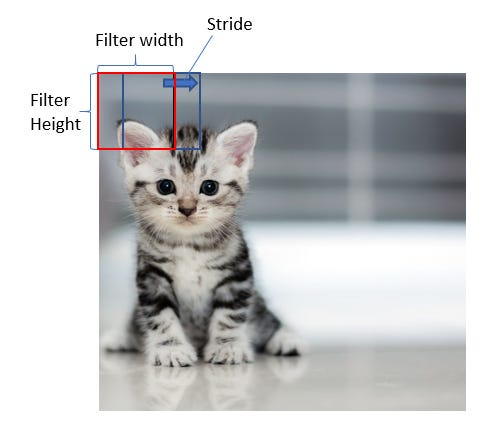A convolution layer with filter width, filter height and stride. Note that while the red and blue boxes look at different areas, they use the same parameters.
• Depth. In our earlier example, we applied just one neuron to identify whether there was a cat or not and share the parameters by applying the same neuron in each quadrant. Suppose we wanted another neuron to identify whether there was a dog or not as well. This neuron would be applied in the same way as the cat-identifying neuron, but have different parameters and therefore a different output for each quadrant. How would this change our parameter and output size? Well, if we had two such neurons, we’d have (128 * 128 * 3 + 1) * 2 = 98,306 parameters. And at the end of Step 4, we’ll have 2 * 2 * 2 = 8 output numbers. The first two terms, 2 * 2, refers to the height and width (of our four quadrant areas) and the last term, 2, refers to the fact that we had two different neurons applied to each quadrant. This last term is what we call depth.

I don’t want to introduce too many concepts all at one go, so let’s give a small quiz to consolidate these concepts.

Suppose we have an image of input size 256 * 256 * 3. I apply a conv layer with filter size 3 * 3, stride 1, and depth 64.

• How many parameters do we have in our conv layer?
• What is the output dimensions of this conv layer?

I encourage you to work this out yourself and don’t scroll down to see the answers! If you wish to check your answers, they are found 5 lines below.

Ok, here are the answers! (I hope you didn’t cheat, but there’s no way I’ll know anyway):

• Number of parameters: We work out the case for depth = 1, since that’s just one neuron applied throughout. This neuron takes in 3 * 3 * 3 (filter size * input channels) features, and so the number of parameters for this one neuron are 3 * 3 * 3 + 1 = 28. Now, we know that depth = 64, meaning there are 64 such neurons. This gives us a total of 28 * 64 = 1,792 parameters.
• Output dimensions: Let’s think of it in the dimension of width first. We have a row of 256 pixels in our original input image. At the start, the center of our filter (what the neuron takes as input) will be at pixel 2, since we have a 3 * 3 filter. Thus, since the leftmost side of the filter will be at pixel 1, the center of the filter will be at pixel 2. This filter moves rightwards by 1 pixel at each time to apply the neuron(s). At the end of all our steps, the center of our filter will be at pixel 255, again because we have a 3 * 3 filter (so pixel 256 will be taken up by the rightmost side of the filter). So given that the center of our filter starts at pixel 2 and ends at 255 while moving 1 pixel each step, the math suggests that we’ve applied the neuron 254 times across the width. Similarly, we’ve applied the neuron 254 times across the height. And since we have 64 neurons doing that (depth = 64), our output dimensions are 254 * 254 * 64.

At this point, you might be wondering: well, what if I wanted output dimensions of 256 * 256 * 64 so that the height and width of our output remains the same as the input dimensions? Here, I introduce a new concept to deal with that exactly:

• Padding. Recall that the center of the 3×3 filter started at pixel 2 (instead of at pixel 1) and ended at pixel 255 (instead of at pixel 256). To make the center the filter start at pixel 1, we can pad the image with a border of ‘0’s, like this: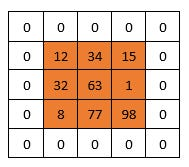This is an example of padding a 3×3 image with a padding border of size 1. This ensures that the center of a 3×3 filter will begin at pixel 1 of the original image.

And with that, we’ve covered exactly what a convolution layer is as used in many cutting-edge systems out there! There is another layer we will introduce, and then we’ll put all the layers together in one big architecture and discuss the intuition behind that!

Summary: A layer common in CNNs in the Conv layer, which is defined by the filter size, stride, depth and padding. The Conv layer uses the same parameters and apply the same neuron(s) across different regions of the image, thereby reducing the number of parameters needed.

The next layer we will go through is called the pooling layer, which corresponds roughly to Steps 4 and 5 in the algorithm laid out at the start. If you recall, we had four numbers in our basic algorithm after applying the conv layer and we wanted it to reduce it to one number. We simply took the four input numbers and output the maximum as our output number. This is an example of max-pooling, which as its name suggests, takes the maximum of the numbers it looks at.

More generally, a pooling layer has a filter size and a stride, similar to a convolution layer. Let’s take the simple example of an input with depth 1 (i.e. it only has 1 depth slice). If we apply a max-pool with filter size 2×2 and stride 2, so there is no overlapping region, we get: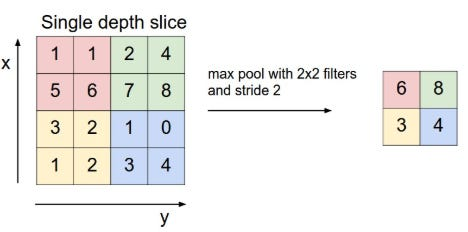Max-pooling with filter 2 and stride 2. Note that a max-pool layer of filter 2 and stride 2 is commonly seen in many models today. Image taken from CS231N notes: http://cs231n.github.io/convolutional-networks/

This max-pool seems very similar to a conv layer, except that there are no parameters (since it just takes the maximum of the four numbers it sees within the filter). When we introduce depth, however, we see more differences between the pooling layer and the conv layer.

The pooling layer applies to each individual depth channel separately. That is, the max-pooling operation does not take the maximum across the different depths; it only takes the maximum in a single depth channel. This is unlike the conv layer, which combines inputs from all the depth channels. This also means that the depth size of our output layer does not and cannot change, unlike the conv layer where the output depth might be different from input depth.

The purpose of the pooling layer, ultimately, is to reduce the spatial size (width and height) of the layers and it does not touch on the depth at all. This reduces the number of parameters (and thus computation) required in future layers after this pooling layer.

To give a quick example, let’s suppose after our first conv layer (with pooling), we have an output dimension of 256 * 256 * 64. We now apply a max-pooling (with filter size 2×2 and stride 2) operation to this, what is the output dimensions after the max pooling layer?

Answer: 128 * 128 * 64, since the max-pool operator reduces the dimensions on the width and height by half, while leaving the depth dimension unchanged.

Summary: Another common layer in CNNs is the max-pooling layer, defined by the filter size and stride, which reduces the spatial size by taking the maximum of the numbers within its filter.

The last layer that commonly appears in CNNs is one that we’ve seen before in earlier parts — and that is the Fully-Connected (FC) layer. The FC layer is the same as our standard neural network — every neuron in the next layer takes as input every neuron in the previous layer’s output. Hence, the name Fully Connected, since all neurons in the next layer are always connected to all the neurons in the previous layer. To show a familiar diagram we’ve seen in Part 1a: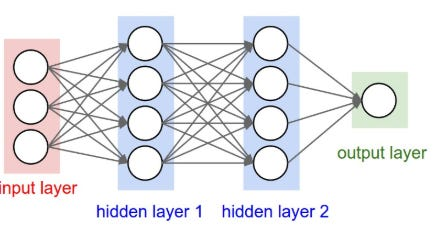Image taken from CS231N Notes (http://cs231n.github.io/neural-networks-1/)

We usually use FC layers at the very end of our CNNs. So when we reach this stage, we can flatten the neurons into a one-dimensional array of features. If the output of the previous layer was 7 * 7 * 5, we can flatten them into a row of 7*7*5 = 245 features as our input layer in the above diagram. Then, we apply the hidden layers as per usual.

Summary: We also typically use our traditional Fully-Connected layers at the end of our CNNs.

Now let’s put them all together. One important benchmark that is commonly used amongst researchers in Computer Vision is this challenge called ImageNet Large Scale Visual Recognition Challenge (ILSVRC). ImageNet refers to a huge database of images, and the challenge of ILSVRC is to accurately classify an input image into 1,000 separate object categories.

One of the models that was hailed at the turning point in using deep learning is AlexNet, which won the ILSVRC in 2012. In a paper titled “The History Began from AlexNet: A Comprehensive Survey on Deep Learning Approaches”, I quote:

AlexNet achieved state-of-the-art recognition accuracy against all the traditional machine learning and computer vision approaches. It was a significant breakthrough in the field of machine learning and computer vision for visual recognition and classification tasks and is the point in history where interest in deep learning increased rapidly.

AlexNet showed that amazing improvements in accuracy can be achieved when we go deep — i.e. stack more and more layers together like we’ve seen. In fact, architectures after AlexNet decided to keep going deeper, with more than a hundred layers!

AlexNet’s architecture can be summarized somewhat as follows: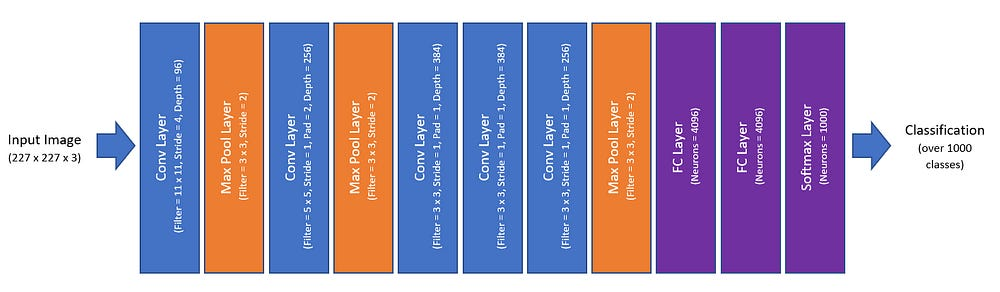A simplistic view of the AlexNet Architecture, where some details have been omitted

As you can see, AlexNet is simply made out of the building blocks of:

• Conv Layers (with ReLU acitvations)
• Max Pool Layers
• FC Layers
• Softmax Layers

These are layers we’ve all seen in one way or another thus far! As you can see, we’ve already covered the building blocks for powerful Deep Learning models and all we need to do is stack many of these layers together. Why does stacking many layers together work, and what is each layer really doing?

We can visualize some of the intermediate layers. This is a visualization of the first conv layer of AlexNet: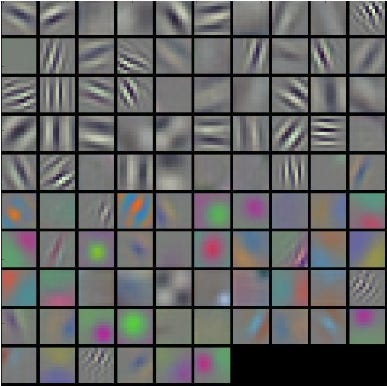A visualization of the first conv layer in AlexNet. Image taken from CS231N notes: http://cs231n.github.io/understanding-cnn/

We can see that at the first few layers, the neural network is trying to extract out some low-level features. These first few layers then combine in subsequent layers to form more and more complex features, and in the end, figure out what represents objects like cats, dogs etc.

Why did the neural network pick out those features in particular in the first layer? It just figured out that these are the best parameters to characterize the first few layers; they simply produced the minimal loss.

Summary: AlexNet was a CNN which revolutionized the field of Deep Learning, and is built from conv layers, max-pooling layers and FC layers. When many layers are put together, the earlier layers learn low-level features and combine them in later layers for more complex representations.

Consolidated Summary: Images are 3-dimensional array of features: each pixel in the 2-D space contain three numbers from 0–255 (inclusive) corresponding to the Red, Green and Blue channels. Often, image data contains a lot of input features. A layer common in CNNs in the Conv layer, which is defined by the filter size, stride, depth and padding. The Conv layer uses the same parameters and apply the same neuron(s) across different regions of the image, thereby reducing the number of parameters needed. Another common layer in CNNs is the max-pooling layer, defined by the filter size and stride, which reduces the spatial size by taking the maximum of the numbers within its filter. We also typically use our traditional Fully-Connected layers at the end of our CNNs. AlexNet was a CNN which revolutionized the field of Deep Learning, and is built from conv layers, max-pooling layers and FC layers. When many layers are put together, the earlier layers learn low-level features and combine them in later layers for more complex representations.

What’s Next: Now that we have looked at how neural networks are applied to images, Part 3 (link) applies neural networks to sequence data, using the example of natural language (a sentence is simply a sequence of words). How would we use neural networks to approach sequences where the input might have varying length?